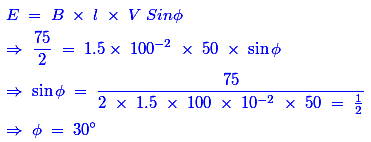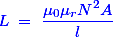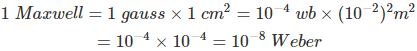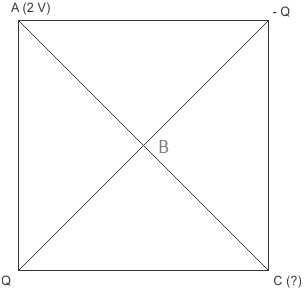# MCQs on Electromagnetic Fields

##### Page 8 of 20. Go to page 1 2 3 4 5 6 7 8 9 10 11 12 13 14 15 16 17 18 19 20
01․ A conductor of length 100 cm moves at right angles to a magnetic field of flux density 1.5 Wb/m2 with a velocity of 50 m/s. The induced emf in the conductor will be
150 V.
50 V.
75 V.
37.5 V.

Given data: Length (l) = 100 cm = 100 × 10-2 m; Flux density (B) = 1.5 Wb/m2; Velocity (V) = 50 m/s; Then, the induced EMF (E) = B × l × V = 100 × 10-2 × 1.5 × 50 = 75 Volts

02․ In above problem the induced emf will be reduced to half if the conductor moves an angle of
30° to the direction of field.
45° to the direction of field.
60° to the direction of field.
75° to the direction of field.

The expression of induced EMF with angle of field is,Hence, the conductor moves an angle of 30° to the direction of field.

03․ Self inductance of magnetic coil is proportional to
N.
1 / N.
N2.
1 / N2.

The expression of self inductance is as follows;Hence, the self inductance of a magnetic coil is directly proportional to N2.

04․ 1 Maxwell is the same as
10-8 weber.
10-8 weber.
104 weber.
10-4 weber.05․ When a magnet is in motion relative to a coil the induced emf does not depend upon
pole strength of the magnet.
motion of the magnet.
resistance of the coil.
number of turns of the coil.

The expression of induced emf in a coils is E = Blv. Where, B is flux density of the magnet, l is length of the coil (if turns of the coil changes, then the length also changes) and v is motion or velocity of the magnet. Hence, the induced EMF does not depend upon the resistance of the coil.magnetic field

06․ A copper disc is rotated rapidly below a freely suspended magnetic needle. The magnetic needle starts rotating with velocity
equal to that of disc and in the same direction.
equal to that of disc and in the opposite direction.
less than that of disc and in the same direction.
less than that of disc but in opposite direction.

When the copper plate is rotated below a freely suspended magnetic needle, there will be a relative velocity between the field of magnetic needle and rotating copper disc. Due to this relative velocity, a circulating current will be induced inside the copper plate. According to the working principle of the induction motor, the needle starts rotating in the same direction but with lesser velocity.

07․ The direction of induced emf can be found by
Laplaces law.
Kirchhoffs voltage law.
Lenzs law.
Flemings right hand rule.

Lenzs law states that the induced current due to the induced emf always flow in such a direction as to oppose the change causing it. Hence, we can find the direction of induced EMF using Lenz’s Law.

08․ An electric field on a place described by its potential V = 20 (r -1 + r-2) where r is the distance from the source. The field is due to
A dipole.
A monopole.
A quardra pole.
Both of A and B.

For a monopole, electric field varies inversely with r2 while its potential varies inversely with r. Similarly for a dipole its electric field varies inversely with r3 and potential varies inversely with r2. In the given expression both terms are present, so this potential is due to both monopole and dipole.

09․ Two point charges (Q and – Q) are situated at the vertex of the square. If potential at another vertex is 2 V, so potential at opposite vertex (diagonally) is
Zero.
1 V.
2 V.
√ 2 V.

Plane between midway Q and -Q i.e one passing through ABC and perpendicular to plane of paper is another equi-potential plane. Hence potential at C = potential at A = 2 V.10․ Inside a hollow conducting sphere
Electric field is zero.
Electric field is a non zero constant.
Electric field changes with distance from the centre of the sphere.
Electric field changes with the magnitude of the charge given to the conductor.

For any point on any concentric sphere inside the conducting sphere, the charge enclosed by the concentric sphere is zero and hence electric field is zero.

<<<678910>>>